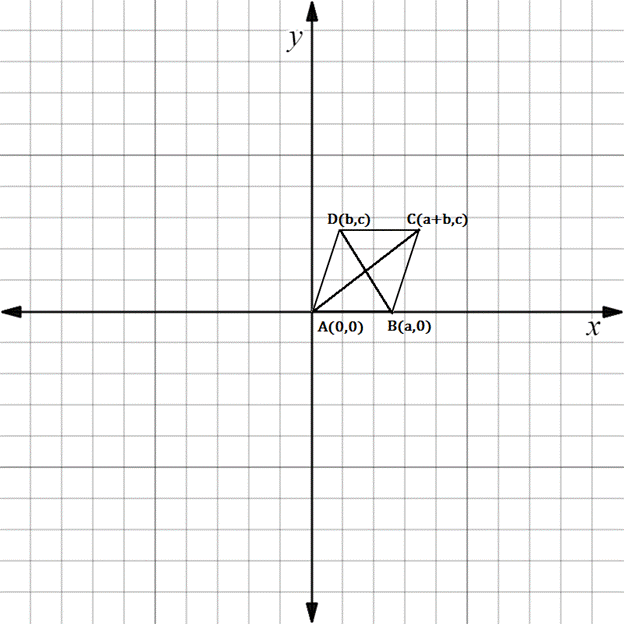Chapter 10.3, Problem 25EElementary Geometry For College St...

7th Edition
Alexander + 2 others
ISBN: 9781337614085

Solutions

Chapter
SectionElementary Geometry For College St...

7th Edition
Alexander + 2 others
ISBN: 9781337614085
Textbook Problem

In Exercises 23 to 28, find the equation (realtionship) requested. Then eliminate fractions and square root radicals from the equation.If ▱ A B C D , suppose that diagonals A C - and D B - are perpendicular. State an equation that relates a ,   b , and c .To determine

To state:

An equation that relates a, b, and c for the given figure.

Explanation

The given figure is shown below.

It is given that AC- and DB- are perpendicular.

Hence, the product of their slopes is -1.

That is mAC·mDB=-1

Finding the slope of AC.

x1,y1=A0, 0

x2,y2=Ca+b, c

Formula for slope of the line containing two points.

Slope is denoted by m.

m=y2-y1x2-x1

Here x1x2

Substituting the x and y co-ordinates.

mAC=c-0a+b-0

Simplifying,

mAC=ca+b

Finding the slope of DB.

x1,y1=Db, c

x2,y2=Ba, 0

Substituting the x and y co-ordinates

Still sussing out bartleby?

Check out a sample textbook solution.

See a sample solution

The Solution to Your Study Problems

Bartleby provides explanations to thousands of textbook problems written by our experts, many with advanced degrees!

Get Started

In Exercises 1-4, simplify the expression by factoring. x23x28x7

Calculus: An Applied Approach (MindTap Course List)

The value of dydx for the function y=x(3x+2).

Mathematical Applications for the Management, Life, and Social Sciences

Evaluate limx1x31x1.

Single Variable Calculus

Differentiate the function. B(y) = cy6

Single Variable Calculus: Early Transcendentals, Volume I

In words, explain what is measured by SS, variance, and standard deviation.

Statistics for The Behavioral Sciences (MindTap Course List)